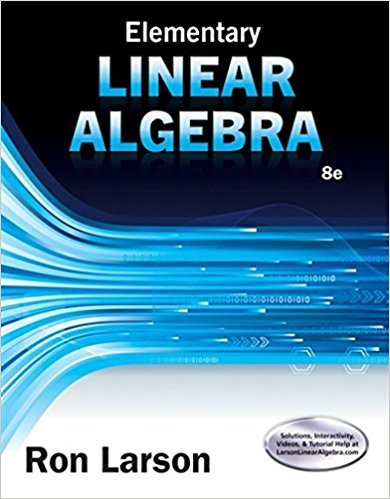×

×

# Solutions for Chapter 1: Systems of Linear Equations## Full solutions for Elementary Linear Algebra | 8th Edition

ISBN: 9781305658004Solutions for Chapter 1: Systems of Linear Equations

Solutions for Chapter 1
4 5 0 343 Reviews
17
5
##### ISBN: 9781305658004

Elementary Linear Algebra was written by and is associated to the ISBN: 9781305658004. This textbook survival guide was created for the textbook: Elementary Linear Algebra, edition: 8. This expansive textbook survival guide covers the following chapters and their solutions. Chapter 1: Systems of Linear Equations includes 144 full step-by-step solutions. Since 144 problems in chapter 1: Systems of Linear Equations have been answered, more than 48511 students have viewed full step-by-step solutions from this chapter.

Key Math Terms and definitions covered in this textbook
• Adjacency matrix of a graph.

Square matrix with aij = 1 when there is an edge from node i to node j; otherwise aij = O. A = AT when edges go both ways (undirected). Adjacency matrix of a graph. Square matrix with aij = 1 when there is an edge from node i to node j; otherwise aij = O. A = AT when edges go both ways (undirected).

• Diagonalization

A = S-1 AS. A = eigenvalue matrix and S = eigenvector matrix of A. A must have n independent eigenvectors to make S invertible. All Ak = SA k S-I.

• Dimension of vector space

dim(V) = number of vectors in any basis for V.

• Factorization

A = L U. If elimination takes A to U without row exchanges, then the lower triangular L with multipliers eij (and eii = 1) brings U back to A.

• Fibonacci numbers

0,1,1,2,3,5, ... satisfy Fn = Fn-l + Fn- 2 = (A7 -A~)I()q -A2). Growth rate Al = (1 + .J5) 12 is the largest eigenvalue of the Fibonacci matrix [ } A].

• Gauss-Jordan method.

Invert A by row operations on [A I] to reach [I A-I].

• Hankel matrix H.

Constant along each antidiagonal; hij depends on i + j.

• Kronecker product (tensor product) A ® B.

Blocks aij B, eigenvalues Ap(A)Aq(B).

• Krylov subspace Kj(A, b).

The subspace spanned by b, Ab, ... , Aj-Ib. Numerical methods approximate A -I b by x j with residual b - Ax j in this subspace. A good basis for K j requires only multiplication by A at each step.

• Linear transformation T.

Each vector V in the input space transforms to T (v) in the output space, and linearity requires T(cv + dw) = c T(v) + d T(w). Examples: Matrix multiplication A v, differentiation and integration in function space.

• Matrix multiplication AB.

The i, j entry of AB is (row i of A)·(column j of B) = L aikbkj. By columns: Column j of AB = A times column j of B. By rows: row i of A multiplies B. Columns times rows: AB = sum of (column k)(row k). All these equivalent definitions come from the rule that A B times x equals A times B x .

• Reduced row echelon form R = rref(A).

Pivots = 1; zeros above and below pivots; the r nonzero rows of R give a basis for the row space of A.

• Semidefinite matrix A.

(Positive) semidefinite: all x T Ax > 0, all A > 0; A = any RT R.

• Similar matrices A and B.

Every B = M-I AM has the same eigenvalues as A.

• Solvable system Ax = b.

The right side b is in the column space of A.

• Spanning set.

Combinations of VI, ... ,Vm fill the space. The columns of A span C (A)!

• Special solutions to As = O.

One free variable is Si = 1, other free variables = o.

• Spectrum of A = the set of eigenvalues {A I, ... , An}.

Spectral radius = max of IAi I.

• Standard basis for Rn.

Columns of n by n identity matrix (written i ,j ,k in R3).

• Stiffness matrix

If x gives the movements of the nodes, K x gives the internal forces. K = ATe A where C has spring constants from Hooke's Law and Ax = stretching.